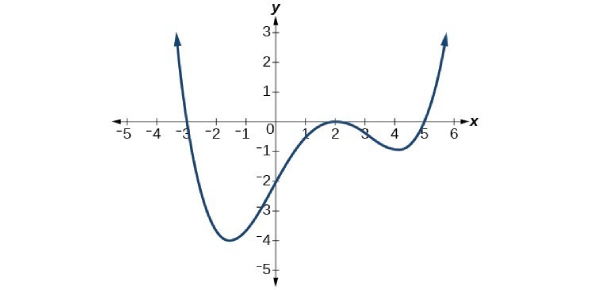# Polynomial Function Quiz Questions And Answers

17 Questions | Attempts: 5643
ShareSettingsHave you studied polynomials well during your math class in school? If you think you know it well, try out polynomial function quiz questions and answers. This quiz is all about polynomial function, with all multiple choice. This will help you become a better learner in the basics and fundamentals of algebra. You could either practice or challenge yourself on this quiz to find out whether you are a pro on the topic or you need more practice. All the best!

• 1.
The graph of a polynomial function is tangent to its?
• A.

Axis

• B.

Y-axis

• C.

X-axis

• D.

Orbit

• 2.
How many possible roots than fourth-degree polynomials can have?
• A.

4

• B.

3

• C.

5

• D.

2

• 3.
Is a linear function a polynomial?
• A.

Sometimes

• B.

Rarely

• C.

Always

• D.

Never

• 4.
What do you call a polynomial of five degrees?
• A.

Pentanomial

• B.

Heptanomial

• C.

Hexanomial

• D.

None of the above

• 5.
What is the graph of a polynomial function?
• A.

Broken

• B.

Continuous

• C.

Sharp end

• D.

Fixed

• 6.
What are the x-intercepts of polynomials?
• A.

(0,a)

• B.

Absolute

• C.

Value of y

• D.

Roots

• 7.
What do you call a polynomial with two as the highest degree 4?
• A.

Linear

• B.

• C.

Ellipse

• D.

Focal point

• 8.
What is the degree of this given, x+2=0?
• A.

0

• B.

1

• C.

2

• D.

3

• 9.
Which is a polynomial of degree 1?
• A.

X+1

• B.

5+5

• C.

Polynomial has no degree 1

• D.

• 10.
What is the constant term in this equation, x+25?
• A.

0

• B.

None

• C.

25

• D.

30

• 11.
How do you define the maximum number of roots in a polynomial?
• A.

Equal to the highest degree of a polynomial

• B.

Given by the polynomial

• C.

Multiplicity

• D.

By the number of roots

• 12.
What theorem states that if  x-c is a factor of f(x), then it becomes f(c)?
• A.

Factor theorem

• B.

Remainder theorem

• C.

Fundamentals of algebra

• D.

Rational root theorem

• 13.
What do you call the shortcut method in dividing polynomials?
• A.

Factor theorem

• B.

• C.

Synthetic division

• D.

Polynomial division

• 14.
What do you call a four-degree polynomial?
• A.

• B.

Linear

• C.

Cubic

• D.

• 15.
What are the roots of G(x)= (x+2)(x-5)(x-1)?
• A.

-2,5,1

• B.

1,2,3

• C.

4,7,5

• D.

X,x,x

• 16.
Is the graph of the polynomial function smooth?
• A.

Yes, and broken

• B.

Yes, continuous, and straight

• C.

Yes, continuous, and curve

• D.

No

• 17.
How do you define the turning points of the graph of polynomial functions?
• A.

(n-1)180

• B.

N+1

• C.

N-1

• D.

N+2

## Related TopicsBack to top
×

Wait!
Here's an interesting quiz for you.